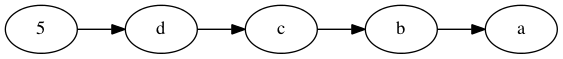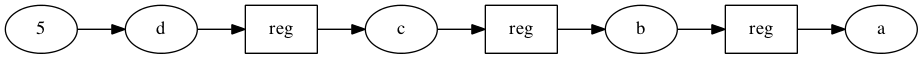# Getting started with PSHDL

In this tutorial I want to give you a short glimpse of how to use PSHDL. So let's start with the most simple program one could imagine.

## A very simple example

``````module de.tuhh.ict.LEDOff {
out bit led=1;
}
``````

In this simple example you can see multiple things. The first one is that each PSHDL file contains a module with code (this is not entirely true, but will work for now). This module has an identifier de.tuhh.ict.LEDOff which contains 2 information. The first one is that the module's name is LEDOff. The other part separated by dots in front of it de.tuhh.ict is its package. Like in Java it should be a unique identifier, which is usually accomplished by using the reverse DNS name of the project. Packages can be used to organize your source code. For now that is enough to know about it.

In-between the curly braces you can put the actual code. The only thing you will find here is a variable which is of type bit and of direction out. PSHDL has 5 builtin primitive types: bit, uint, int, bool, string.

• bit is used for variables that do not have an arithmetic meaning. Those could for example be control variables, clocks, resets etc.
• uint is an unsigned integer. That is, it can not be negative and in arithmetic operations it is considered positive.
• int is a signed integer. It may become negative, if used together with uint in arithmetic operations, the other side is always converted to int as well.
• bool is for storing the result of comparisons. It can only be used with the boolean operators || and &&. It can also be used in if conditions.
• string can be useful for configuration. It can only be used to be passed to functions or be compared with other strings.

### What does this little example do?

Well, first of all it describes a module which has one port (ports are variables that have a direction attached to them). This port is always high as it is initialized with a 1. If you would leave the initialization away, you would get an out port that is always 0.

## More LEDs!

Assume we would want to drive 5 LEDs instead of just one. We could accomplish this by writing:

``````module de.tuhh.ict.LEDOff {
out bit<5> led=0xA;
}
``````

What you can see here is that literals can be written in multiple ways:

• As plain integer
• In hex with a preceding 0x
• As binary with a preceding 0b

For added readability you can use underscores to separate nibbles, decimals, or whatever seems reasonable for you. Literals always adopt the size of their assignment target or other operand.

What you can also see is that primitives can have a width. That is, the variable led contains 5 bits. Width are written in-between '<' '>'. Those can be literals or constant expressions. If you do not specify any width the following defaults are used:

• bit is 1 bit
• uint is 32 bit
• int is 32 bit

## Timing model

The most important thing to understand when using PSHDL is that it is not a sequential programming language like C or Java. It is a hardware description language and everything happens in parallel. To understand this here a little example:

``````module de.tuhh.ict.Timing {
out uint a=1,b=2,c=3,d=4;
a=b;
b=c;
c=d;
d=5;
}
``````

If this were Java you would expect the following:

• a is first 1, then 2.
• b is first 2, then 3.
• c is first 3, then 4.
• d is first 4, then 5.

This is not true in PSHDL. Every variable is 5. You can think of variables (that are not registers) as wires. A wire does not consume any time to transport its value.So the order of writing to a variable itself is not important. What is important however is the order of assignment to the same variable.

``````module de.tuhh.ict.Timing {
out uint a=1,b=2;
if (b==2)
a=5;
a=6;
}
``````

No matter what value b actually has, the last evaluated assignment to a is 6. Thus it is always 6. This is different from this:

``````module de.tuhh.ict.Timing {
out uint a=1,b=2;
a=6;
if (b==2)
a=5;
}
``````

The if statement is true and thus the assignment a=5 is the last assignment to a. Now a is 5.

But what about registers? Let's take a look at the initial example but with registers.

``````module de.tuhh.ict.Timing {
out register uint a=1,b=2,c=3,d=4;
a=b;
b=c;
c=d;
d=5;
}
``````Before the first clock the reset value of the register is used which is (unless you specify it differently with the resetValue= parameter of registers) 0.

On the first clock the assignment of d=5 becomes visible, that is, the input of the register has been 'registered' and is now visible at the output. c,b and a however still saw the 0 as input and thus are 0 too.

cycle a b c d
0 0 0 0 0
1 0 0 0 5
2 0 0 5 5
3 0 5 5 5
4 5 5 5 5

Let us expand the little code snippet to something that blinks.

``````module de.tuhh.ict.LEDOff {
out bit<5> led=counter{6:1};
register uint<7> counter;
counter+=1;
}
``````

So much new stuff here, let's start with the register keyword. One of the advantages of PSHDL is that the most important concept of synchronous designs is easy to use, the register. If you want to infer one, you simply put the register keyword in front of a variable. But where is the clock and reset and what does it do?

Declaring a register without any further arguments does the following:

• It is triggered on the rising edge of \$clk
• It is reset synchronously with a 1 on \$rst
• Upon reset it is set to 0

Each of these assumptions can be modified by providing arguments to the register. See the documentation for further information. But what is \$clk and \$rst? Those are two special 1 bit variables that you don't have to declare. If nothing else is declared (see annotations for specifying variables to use as \$clk, \$rst) using one of these variables will create a in bit clk or respectively in bit rst port. So in the end this little code snippet will expand to:

``````module de.tuhh.ict.Blink {
out bit<5> led=counter{6:1};
@clock in bit clk;
@reset in bit rst;
register(clock=clk, reset=rst) uint<7> counter;
counter+=1;
}
``````

As we can see, the width of the counter is 7, while we only have 5 LEDs. So we need to throw away some bits. With '{' and '}' you can access bits. In PSHDL the {0} bit is always the LSB and the highest bit that can be accessed is {width of type-1} which is the MSB. You can also access a range of bits with {from:to}. The result of accessing bits is of type bit< (from-to) + 1 >. The from value has to be greater or equal to the to value.

PSHDL also comes with a simplified syntax for a=a <op> X. Those infix operations are well known from languages like C and Java and do exactly the same. Note however that those can only be used by registers as they would otherwise form a combinatorial loop.

## Modularize it

Well, that counter thing seems like something that could be extracted into its own entity and provide a nice clock divider. So in one file we can write:

``````module de.tuhh.ict.counters.Counter {
param uint USEBIT=7;
out register bit newClk=counter{USEBIT-1};
register uint<USEBIT> counter=counter+1;
}

import de.tuhh.ict.counters.*;
Counter div(USEBIT=20);
div.clk=\$clk;
div.rst=\$rst;
out bit led=div.newClk;
}
``````

The most obvious thing to see here is that you can have multiple modules within one file. Here we have two de.tuhh.ict.counters.Counter and de.tuhh.ict.SlowBlink. The SlowBlink module implicitly imports all modules/interfaces (we will come later to those) of the package de.tuhh.ict. The counter however is in a different package and thus needs to be imported. This allows it to instantiate the Counter with its simple name. An alternative would have been to use the full name:

``````de.tuhh.ict.counters.Counter div;
``````

As you can see, you do not need to specify the parameter value and simply use its default value (7). Accessing the ports is as simple as instanceName.port

## Instantiate VHDL Entities

While it is fun to have everything written in PSHDL, it is also quite unrealistic. Most code is written in Verilog and VHDL. So PSHDL attempts to make using external IPCores as painless as possible.

``````interface VHDL.work.Counter {
param uint USEBIT=7;
in bit clk;
in bit rst;
out bit newClk;
}

import VHDL.work.*;
Counter div(USEBIT=20);
div.clk=\$clk;
div.rst=\$rst;
out bit led=div.newClk;
}
``````

The interface declaration that you see here represents the entity declaration that would be created for the Counter. You can automatically create those interface declarations from VHDL files, but not yet in the web version. There is virtually no difference between instantiating a module and instantiating an interface. The interface could also be a netlist, a Verilog file or anything else that can be instantiated. It is up to you to let the synthesis tool find that entity.

## State-machines

Describing a state-machine is quite simple:

``````module de.tuhh.ict.Statemachine{
enum States={IDLE, WAITING}
register enum States state;
switch (state) {
case States.IDLE:
state=States.WAITING;
case WAITING:
state=IDLE;
default:
state=States.IDLE;
}

out bit x=0;
if (state==States.IDLE)
x=1;
}
``````

The first thing you might notice is that there is a declaration of an enum type that will be known as States. To be a bit more specific, its fully qualified name is de.tuhh.ict.Statemachine.States as it is contained within a module. You could also declare an interface in a module.

When you want to create a variable that is of type enum you have to tell the compiler so by putting the enum keyword before the Type name. The variable can now become any value of that enum and also is a register. This makes describing the next state quite simple, you just set the state variable. But note that when you write:

``````register enum States state=IDLE;
``````

you need to write the state register in any switch case, otherwise it would jump to IDLE if nothing else is written to it.

Whenever the compiler is able to determine that the type can only be of a certain enum, you can leave the Type away, that is instead of writing States.IDLE you can write IDLE.

## Generators

Most systems contain a processor these days, which is why some of those can also be found in many FPGA designs. Those are usually connected to the fabric with some kind of bus. PSHDL makes it easy to use those busses, or use generated entities in general with the generator concept. The following example creates everything you need to get a fully usable IPCore that can be instantiated from within Xilinx Platform Studio:

``````module BUSTest{
include Bus bus=generate axi(regCount=3);
bus.regs=(uint<32>)bus.regs+(uint<32>)bus.regs;
}
``````

This simple example declares an interface that is named Bus and and a variable bus. The interesting part is that this interface does not exist yet. It is generated during compilation by the generator axi. The include keyword indicates that the generated interface is merged into the module. Including has the advantage that all interface ports become ports of the module BUSTest.

The newly created Bus has a port regs that can be used to read and write registers. Those registers can then be accessed via the axi bus at a certain memory address. It is important to understand that including the generated interface may lead to additional variable declarations within the module.

To be continued...